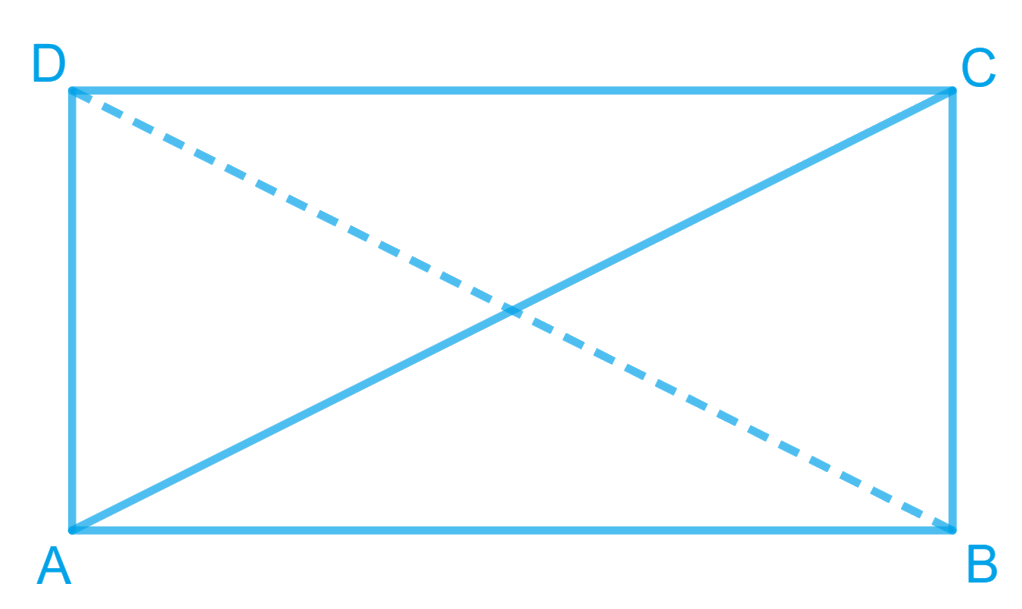In the verge of coronavirus pandemic, we are providing FREE access to our entire Online Curriculum to ensure Learning Doesn't STOP!

# Ex.8.1 Q8 Quadrilaterals Solution - NCERT Maths Class 9

Go back to  'Ex.8.1'

## Question

$$ABCD$$ is a rectangle in which diagonal $$AC$$ bisects $$\angle A$$as well as $$\angle C$$. Show that:

(i) $$ABCD$$ is a square

(ii) Diagonal $$BD$$ bisects $$\angle B$$ as well as $$\angle D$$.

Video Solution
Ex 8.1 | Question 8

## Text Solution

What is known?

$$ABCD$$ is a rectangle in which diagonal $$AC$$ bisects $$∠A$$ as well as $$∠C.$$

What is unknown?

How we can show that

(i) $$ABCD$$ is a square

(ii) Diagonal $$BD$$ bisects $$∠B$$ as well as $$∠D.$$

Reasoning:

We can use angle bisector property and isosceles triangle property to show given rectangle as square and alternate interior angles property to show BD bisects angles B and D.

Steps:(i) It is given that $$ABCD$$ is a rectangle.

\begin{align}\angle {\rm{A}} &= \angle {\rm{C}}\\\Rightarrow \frac{1}{2}\angle {\rm{A}} &= \frac{1}{2}\angle {\rm{C}}\\\Rightarrow \angle \rm DAC &= \frac{1}{2}\angle {\rm{DCA }} \{\text{AC bisects }}&\angle {\rm{A \,and }}\angle\,\, {\rm{C}})\end{align} \(CD = DA (Sides opposite to equal angles are also equal)

However, $$DA = BC$$ and $$AB = CD$$

(Opposite sides of a rectangle are equal)

$$AB = BC = CD = DA$$

$$ABCD$$ is a rectangle and all the sides are equal.

Hence, $$ABCD$$ is a square.

(ii) Let us join $$BD$$

In $$\Delta {BCD}$$

$$BC=CD$$ (Sides of a square are equal to each other)

$$\angle CDB=\angle CBD$$(Angles opposite to equal sides are equal)

However, $$\angle CDB = \angle ABD$$ (Alternate interior angles for $$AB \;||\; CD$$)

$\angle CDB = \angle ABD$

$$BD$$ bisects $$\angle B$$

Also, $$\angle CDB = \angle ABD$$ (Alternate interior angles for $$BC \;||\; AD$$) $$\angle CDB = \angle ABD$$

$$BD$$ bisects $$\angle D$$ and $$\angle B$$.

Video Solution